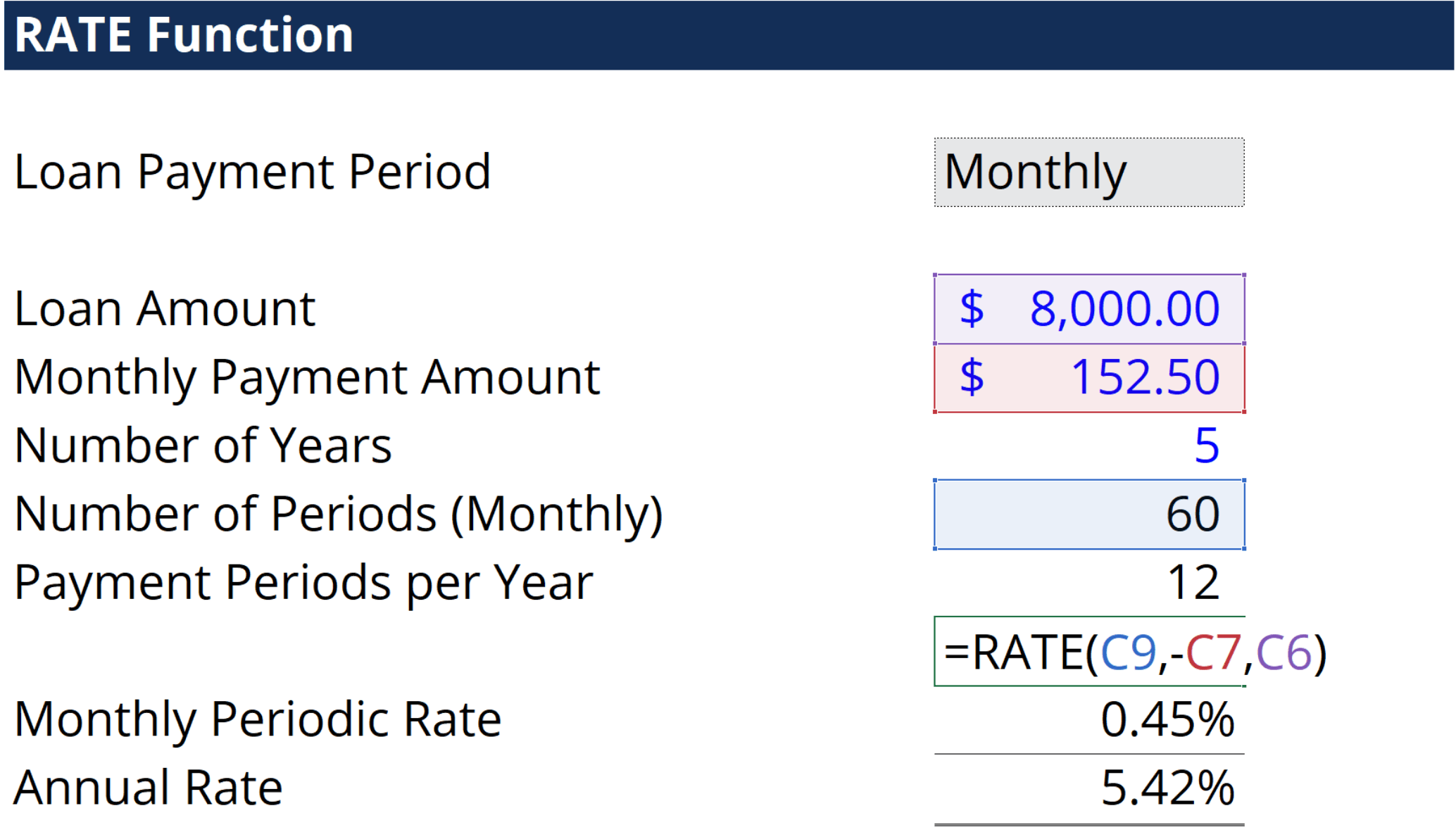# RATE Function

Calculates the interest rate per period of a year

## What is the RATE Function?

The RATE function is an Excel Financial function that is used to calculate the interest rate charged on a loan or the rate of return needed to reach a specified amount on an investment over a given period.

For a financial analyst, the RATE function can be useful to calculate the interest rate on zero coupon bonds.

### Formula

=RATE(nper, pmt, pv, [fv], [type], [guess])

The RATE function uses the following arguments:

1. Nper (required argument) – The total number of periods (months, quarters, years, etc.) over which the loan or investment is to be paid.
2. Pmt (required argument) – This is the payment for each period. This number must be unchanged over the life of the loan. Pmt includes principal and interest but no other fees or taxes. If pmt is omitted, fv must be inputted.
3. PV (required argument) – The present value of all future payments; what all future payments would be worth in the present.
4. FV (optional argument) – This is the future value that is the goal of the investment. This value is what we aim to have after the last payment is made. If we omit fv, it is assumed to be 0 (the future value of a loan, for example, is 0) and we must include a pmt argument instead.
5. Type (optional argument) – Determines how the formula will consider the due dates for payments. If type is omitted or 0 is inputted, payments are due at period end. If 1 is inputted, payments are due at period beginning.
6. Guess (optional argument) – Our guess of what the interest rate should be. This provides a start point for the RATE function so that it may converge on an answer easier before reaching 20 iterations.
1. When omitted, RATE assumes the guess to be 10%.
2. If RATE does not converge, attempt other values for this input.

### How to use the RATE Function in Excel?

As a worksheet function, the RATE function can be entered as part of a formula in a cell of a worksheet. To understand the uses of the function, let us consider an example:

#### Example – Using the RATE function

Suppose the following situation. You are taking out a loan of \$8,000. The loan term is 5 years and payments are made monthly. Loan payments are \$152.50. What would the interest rate be for this loan? We can use the RATE function in Excel to determine this.With this, we can determine that the annual interest rate for this loan is 5.42%. You will notice that cell C7 is set to negative in the formula. This is because this calculation is from the perspective of the person taking on the loan. Translating this formula, C7 is the monthly payment amount. It is the cash outflow that the individual must pay every month. Therefore, he is losing C7, thus causing it to be a negative number.

If this situation was to be calculated from the perspective of the bank issuing the loan to the borrower, C7 would instead be a positive and C6 would be the negative number. This is because the loan would be gaining C7 on a monthly basis (cash inflow of monthly payments). It would also be losing the initial loan amount, thus causing C6 to be the negative number instead.

### Notes to Remember About the RATE Function:

#1. #NUM! error – If the results of RATE do not converge to within 0.0000001 after 20 iterations, RATE returns the #NUM! error value. This may be caused by failure to use the negative or positive sign with regards to cash flow conventions. This is where the above example comes in. You will notice that C7 is negative because it is a cash outflow of monthly payments. C6 is positive because it is a cash inflow of the initial loan amount. If there was no negative in C7, this formula would output a #NUM! error.

Sometimes it is necessary to provide a guess for the formula to work. Because the answers of RATE must converge within 20 iterations, providing a guess allows it to start its iterations closer to the correct answer, potentially allowing it to find the answer before 20 iterations have occurred.

#2. #VALUE! error – Appears when any of the arguments are non-numeric.

#3. The RATE function will output the rate for the period that the loan payments are in. For example, if you are calculating an interest rate for a loan with monthly payments like above, the interest rate calculated by the RATE function will be a monthly interest rate. In order to find the annual interest rate, you will need to multiply the quarterly interest rate outputted by the RATE function by 12.

#4. The RATE function also requires the ‘nper’ argument to be the total number of loan payment periods. Ensure that this number is inputted in the correct units. Using the example above, the nper inputted is 60 because it is 5 years multiplied by 12 months per year.

Thanks for reading CFI’s guide to important Excel functions! By taking the time to learn and master these functions, you’ll significantly speed up your financial analysis. To learn more, check out these additional CFI resources: